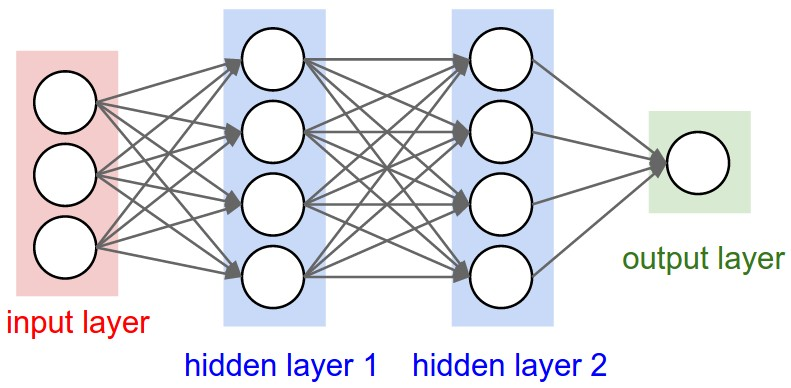# Marko Jerkić

Learner's Deep Learning Blog

## How To Build A Simple Prediction App Using Tensorflow

In this blog post, I’ll be showing you how to create a simple prediction app using Tensorflow. Bear in mind that I am only a beginner and I’m just learning. Please do not take this example as the guideline for how this supposed to be done.

For more reliable data about deep learning, check out some of my newer post.

//NOTE: This simple example is going to use body measurements like weight, height etc. This is not going to be an image recognition app. We’ll get to that eventually.

## Step 1: Dependencies

For this simple example, the only two dependencies that we’re going to use are numpy and Tensorflow.

## Step 2: Create data

In a perfect world, we would have access to  a huge database with many body measurements. But this is not a perfect world.

Because of that, we’re going to need to create our own data.

For this example, we are going to create three sets of data: training data, validation data and test data.

https://gist.github.com/markojerkic/62f984c995932acbee9954b2387a4e15

Feel free to add a few more examples. I added only five examples, so mine results are not very accurate. This example is not intended to create a useful application, but to show you how to create a simple Tensorflow classifier.

Also I am Croatian and we use the metric system so the measurements are in kilograms, centimeters etc.

## Step 3: Create a Tensorflow graph

https://gist.github.com/markojerkic/73e95b01b2989e8052d50fa3e58cebdf

A Tensorflow graph is used to create a data flow graph. A data flow graph consists of nodes and lines which connects them.This image above shows a visualization of a neural network’s data flow graph. Each of those graphs represents a computation node.

These four lines of code above create tensors. Tensors are the main data structures which Tensorflow uses, hence the name.

After that, on lines 8 and 9, we create weights and biases for each node.

We give weights a random value and set biases to zero.

Next we calculate logits. Logits are the essence of neural networks. Logits are the result of the sum of the multiplied weights and  dataset with the biases.

Loss is the difference between the predicted result and the true value. The goal is to get the mean as close to zero as possible.

Now we create a optimizer. We’ll use a gradient descent optimizer with the learning rate of 0.5 and we call the minimize function passing loss as the parameter.

## Step 4:  Train the model

https://gist.github.com/markojerkic/26799382afdb7411b3086cc391bffcb6

The first function returns the percentage of accuracy.

Now we create a session. We initialize our variables (the dataset and label tensors). We’ll run this 5000 times.

The run functions return exactly what we have passed to it – optimizer, loss and predictions.x

Next, every 100 steps we print out accuracy of the prediction. Don’t worry if the prediction percentage is low. Most probably it’s because our dataset is really small.

## Entire app code base

https://gist.github.com/markojerkic/526af6374c1c448c1ca4803c01703209